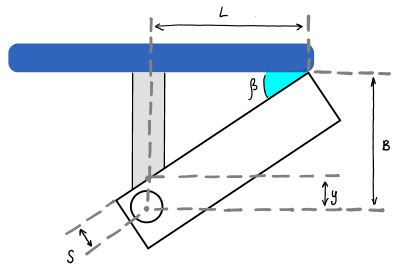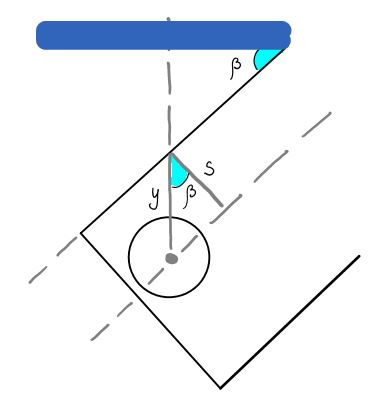motors_init

# Initialization - Zero reference

During homing the robot has to set his zero position. Zero position is choosen as the point where all three arms of the robot are in horizontal position.

### Mechanical Limits of the Axis Motors - Upper limitUpper limit calculation shown below Upper limit calculation detail

The mechanical dimensions are given by L = 0.0175m, B = 0.015m, s = 0.006m. The upper limit can be calculated as follows:

```(1) tan(β) = sin(β) / cos(β) = (B - y) / L
(2) cos(β) = s / y```

Substitute (2) into (1) to get:

`(3) L * sin(β) - B * cos(β) = -s`

Using following trigonometric formulas in (3):

```(4) t = tan(β / 2)
(5) sin(β) = 2 * t / (1 + t^2)
(6) sin(β) = (1 - t^2 / (1 + t^2)```

`(7) (s + B) * t^2 + 2 * L * t + (s - B) = 0`

Solutions are:

```t1 = 0.2264 -> β = 0.445
t2 = -2.1157 (neg. solution)```

Hence, the maximum angle the axis can turn is π/2 + β ≅ 2.016.

### Mechanical Limits of the Axis Motors - Lower limit

For the lower limit of the Axis Motor the angle γ is measured to be about 5°.